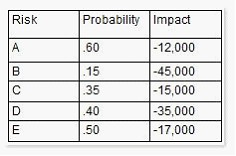# how much will Donna have left in the risk contingency reserve if none of the risk events have happened?

Donna is the project manager of the QSD Project and she believes Risk Event D in the following figure is likely to happen.If this event does happen, how much will Donna have left in the risk contingency reserve if none of the risk events have happened?A.
\$41,700

B.
\$6,700

C.
\$35,000

D.
\$14,000

Explanation:

To answer this question, you’ll first need to calculate the contingency reserve. Contingency reserves are estimated costs to be used at the discretion of the project manager to deal with anticipated, but not certain, events. These events are “known unknowns” and are part of the project scope and cost baselines. The contingency reserve is calculated by multiplying the probability and the impact for the risk event value for each risk event. The sum of the risk events equals the contingency reserve for the project. The sum of the risk events equals the contingency reserve for the project. In this question, the value is \$41,700. If Risk D happens, it’ll cost the project \$35,000. The difference of \$35,000 and \$41,700 is \$6,700.

Answer option C is incorrect. This is the impact of Risk Event D.

Answer option D is incorrect. \$14,000 is the risk event value of Risk Event D.

Answer option A is incorrect. \$41,700 is the amount of the contingency reserve.

Reference: Chapter 11. A Guide to the Project Management Body of Knowledge, (PMBOK Guide), Fourth Edition, ISBN:9781933890517, Section 11.6.2.5.